# Statistics Class 9 Notes: Chapter 14

## Introduction to Statistics

### Introduction to Statistics

– A study dealing with the collection, presentation and interpretation and analysis of data is called as statistics.

### Data

• Facts /figures numerical or otherwise collected for a definite purpose is called as data.
• data collected first-hand data:- Primary
• Secondary data: Data collected from a source that already had data stored

### Frequency

– The number of times a particular instance occurs is called frequency in statistics.

### Ungrouped data

Ungrouped data is data in its original or raw form. The observations are not classified in groups.

### Grouped data

In grouped data, observations are organized in groups.

### Class Interval

• The size of the class into which a particular data is divided.
•    E.g divisions on a histogram or bar graph.
• Class width = upper class limit – lower class limit

### Regular and Irregular class interval

• Regular class interval: When the class intervals are equal or of the same sizes.
• E.g 0-10, 10-20, 20-30….. 90-100
• Irregular class interval: When the class intervals are of varying sizes.
• E.g 0-35, 35-45, 45-55, 55- 80, 80-90, 90-95, 95-100

### Frequency table

– A frequency table or distribution shows the occurrence of a particular variable in a tabular form.

### Sorting

• Raw data needs to be sorted in order to carry out operations.-
• Sorting ⇒ ascending order or descending order

### Ungrouped frequency table

– When the frequency of each class interval is not arranged or organised in any manner.

### Grouped frequency table

– The frequencies of the corresponding class intervals are organised or arranged in a particular manner, either ascending or descending.

To know more about Statistics, visit here.

## Graphical Representation of Data

### Bar graphs

Graphical representation of data using bars of equal width and equal spacing between them (on one axis). The height

 Savings (in percentage) Number of Employees (Frequency) 20 105 30 199 40 29 50 73 Total 400

The data can be represented as: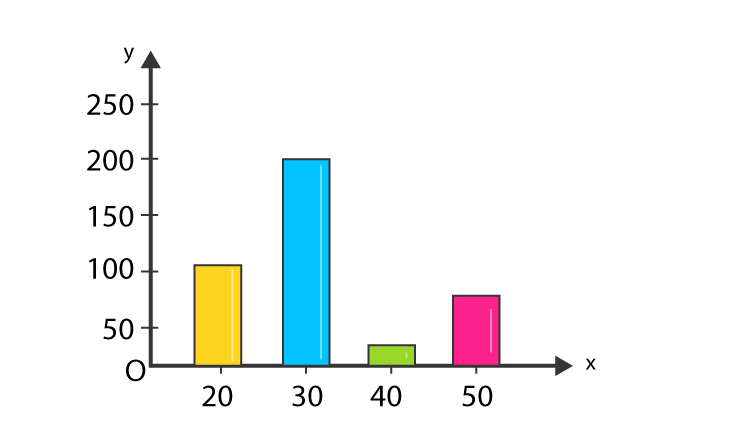#### For More Information On Bar Graphs And Double Bar Graphs, Watch The Below Videos.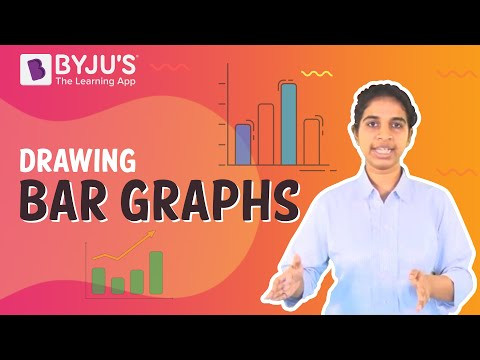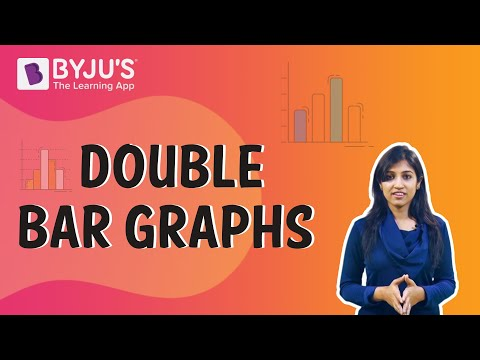To know more about Graphical Representation of Data, visit here.

### Variable being a number

• A variable can be a number such as ‘no. of students’ or ‘no. of months’.
• Can be represented by bar graphs or histograms depending on the type of data.

Discrete bar graphs
Continuous Histograms

### Histograms

• Like bar graphs, but for continuous class intervals.
• Area of each rectangle is ∝ Frequency of a variable and the width is equal to the class interval.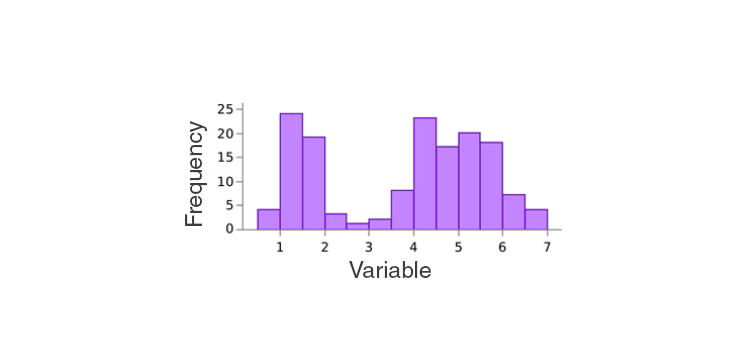To know more about Histogram, visit here.

### Frequency polygon

• If the midpoints of each rectangle in a histogram are joined by line segments, the figure formed will be a frequency polygon.
• Can be drawn without histogram. Need midpoints of class intervals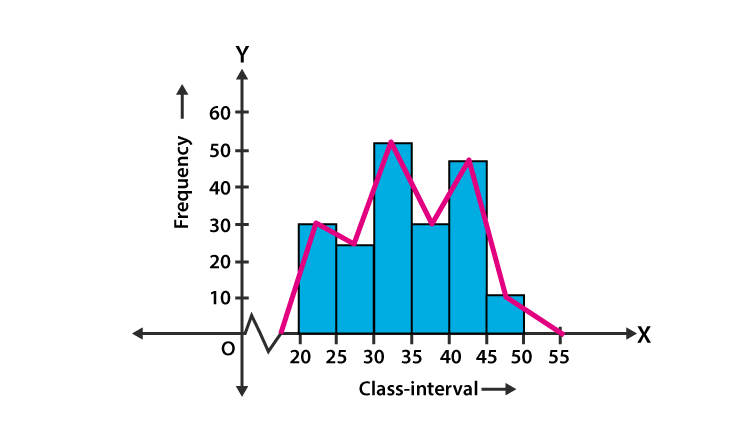To know more about Frequency Polygon, visit here.

### Midpoint of class interval

The midpoint of the class interval is called a class mark
Class mark = (Upper limit + Lower limit)/2

### Equality of areas

– Addition of two class intervals with zero frequency preceding the lowest class and succeeding the highest class intervals enables to equate the area of the frequency polygon to that of the histogram(Using congruent triangles.)

## Measures of Central Tendency

### Average

– The average of a number of observations is the sum of the values of all the observations divided by the total number of observations.

### Mean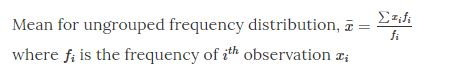### Mode

• The most frequently occurring observation is called the mode.
• The class interval with the highest frequency is the modal class

### Median

• Value of the middlemost observation.
• If n(number of observations) is odd, Median =[(n+1)/2]th observation.
• If n is even, the Median is the mean or average of (n/2)th and [(n+1)/2]th observation.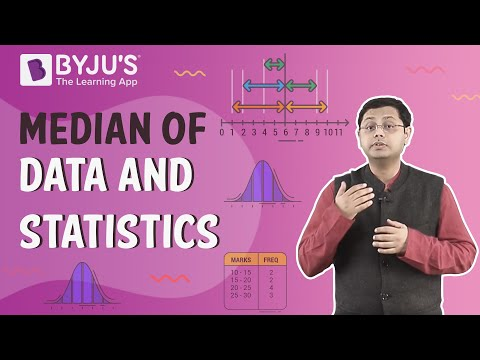To know more about Measures of Central Tendency, visit here.

## Frequently asked Questions on CBSE Class 9 Maths Notes Chapter 14: Statistics

### What is a ‘Frequency polygon’?

A frequency polygon is a line graph of class frequency plotted against class mid-point.

### What is a ‘Median’?

The median is the middle number in a sorted, ascending or descending, list of numbers.

### What are some of the uses of ‘Statistics’?

Statistics is used in the tudy and manipulation of data, including ways to gather, review, analyse and draw conclusions from data.